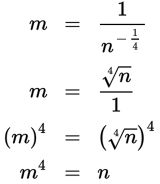# SAT Math Multiple Choice Question 339: Answer and Explanation

### Test Information

Question: 339

9. If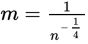, where both m > 0 and n > 0, which of the following gives n in terms of m?

• A.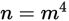• B.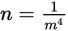• C.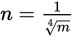• D.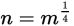Explanation:

A

Difficulty: Medium

Category: Passport to Advanced Math / Exponents

Strategic Advice: When you write an equation in terms of a specific variable, you are simply solving the equation for that variable. In this question, you'll need to relate fractional exponents to radicals and understand how to use negative exponents. Be careful-you're not just rewriting the equation, you're also solving it for n.

Getting to the Answer: Raising a quantity to the one-fourth power is the same as taking its fourth root. Applying a negative exponent to a quantity is the same as writing its reciprocal. Rewrite the equation using these properties, and then solve for n using inverse operations. Note that the inverse of taking a fourth root of a quantity is raising the quantity to the fourth power.